Himanshi Singh — March 3, 2021

## Introduction

Perceptron is one of the most fundamental concepts of deep learning which every data scientist is expected to master. It is a supervised learning algorithm specifically for binary classifiers.

Note: If you are more interested in learning concepts in an Audio-Visual format, We have this entire article explained in the video below. If not, you may continue reading.

In this article, we will develop a solid intuition about Perceptron with the help of an example. Without any further delay, let’s begin!

Before you continue, I recommend you check out the following article-

Deep Learning 101: Beginners Guide to Neural Network

## Understanding Perceptron with an Example

Let’s start with a simple example of a classification problem. Our aim here is to predict whether the loan should be approved or not, depending on the salary of a person.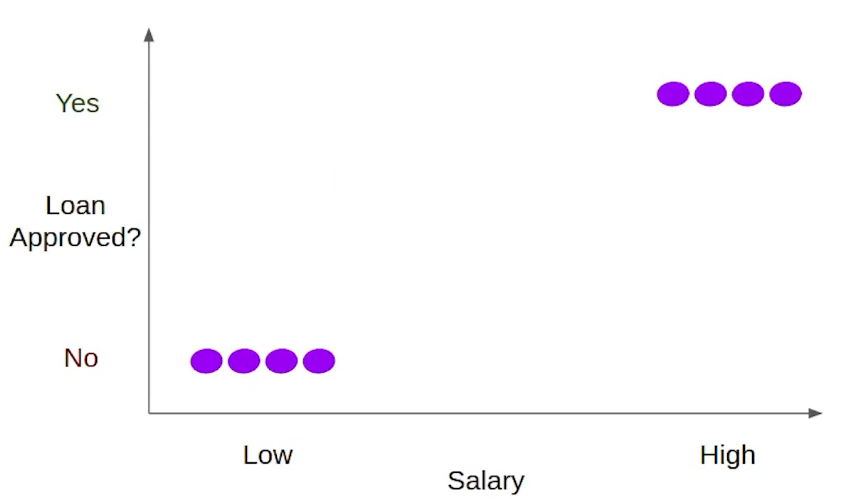In order to do that will need to build a model that takes the salary of the person as an input and predicts whether the loan should be approved for the person or not.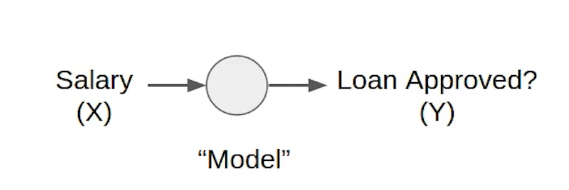Suppose your bank wants to reduce the risk of loan default and hence decides to roll out loans to only such individuals who have a monthly salary of 50000 and above.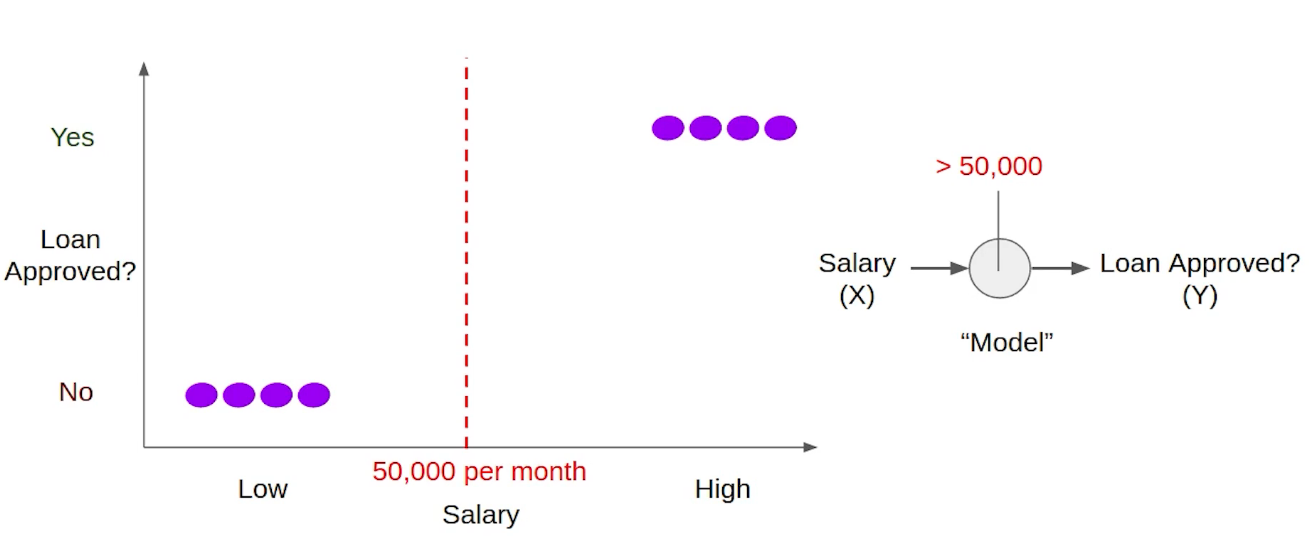In this case, we want our model to learn to check whether the salary input which is represented as X here, is greater than 50000 or not. Here are the tasks we want our model to perform-

• The first thing is it should take in the salary as input.
• Next, it has to check whether the given salaries are greater than 50,000 or not.
• And if the salary’s more than 50,000 only then give output as “Yes”.

Effectively, this model takes in some input, processes it, and generates an output. This is similar to what happens in a biological neuron: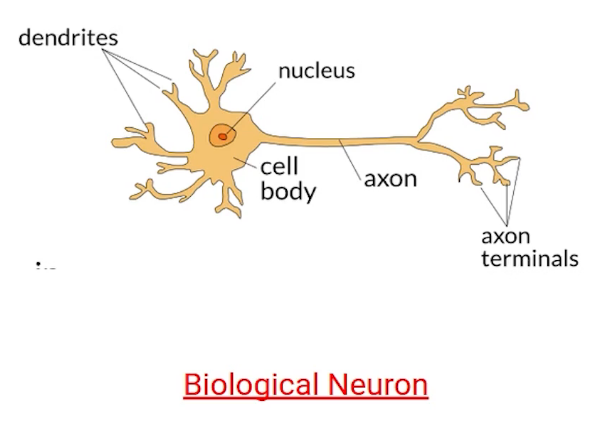It takes the input to the dendrite, processes the provided information, and generates the output. You can see the similarity right? Thus the model that we’re talking about can also be called a Neuron.

Now coming back to our loan example. Let’s have a closer look at each of these tasks, starting from the input. We have a single input which is salary but in general, we can have multiple features just like the applicant’s salary, his/her father’s salary, spouse’s salary that can be deciding factors to approve the Loan. And our neurons will take in all of these features as input in order to make decisions. This is to say that the neuron will have multiple inputs. This is similar to the multiple dendrites that we saw in the biological neuron.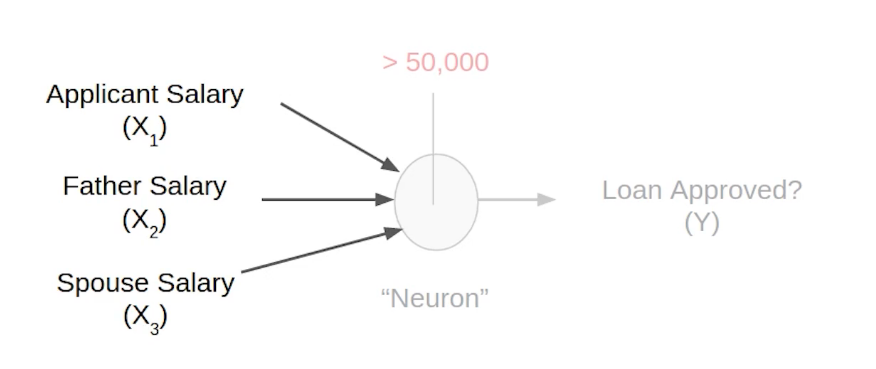Now that there are multiple salary features about the person we’ll take all of them into account as they represent the total income of the household. We can sum them up and check if the total income of the household crosses the threshold or not.

So, the Total Income = Applicant Salary(X1) + Father Salary(X2) + Spouse Salary(X3)

We need to compare this Total Income with the Threshold. Here is the equation representing the same:We have X1, X2, and X3 as input features and we want to check if their sum crosses the particular threshold, which is 50000 in our case. Now if you bring this threshold to the left side of the equation it will become something like this:and if we represent this whole quantity which is “- threshold” with a new term Bias, the updated equation would look something like:this will have the sum of four quantities which are X1, X2, and X3, and note that the bias is actually “- threshold”.

Now this quantity which is Bias, although we have selected it arbitrarily here, it is actually something that neuron learn from the underlined data. If the input exceeds the magnitude of the bias, we want the neuron to give the output as “YES”. That means the loan can be approved by this person. This event is known as the firing of a neuron. If want to write this relationship using equations, we can use the  following equations: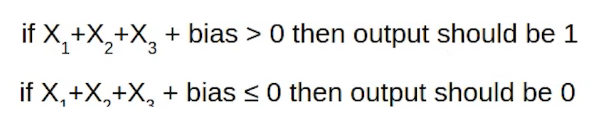We will say that output should be 1 when this equation is true and output should be 0 in all other cases. These two equations can be represented in the form of a function. Let’s see how: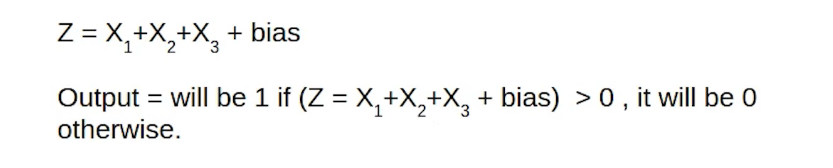So here we have the sum of the features X1, X2, X3, and bias represented as Z and we want our output to be 1 if the Z is greater than 0, otherwise 0.

So we can use a Step Function here and this is the graph of the step function: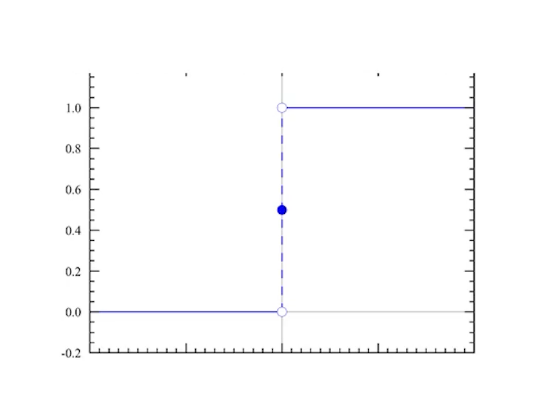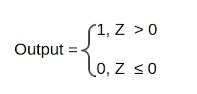It basically gives us the output 1 for any value greater than zero and gives an output 0 for any value less than zero. So in order to find output, we will apply the step function on Z here. We have denoted this step function as following;This step function in this case is used to scale the output of the neuron and in Deep Learning we have an option of choosing such functions to apply to the output of the neurons. They are known as Activation Functions. So when we use the step function as the activation function for a neuron it is called a Perceptron.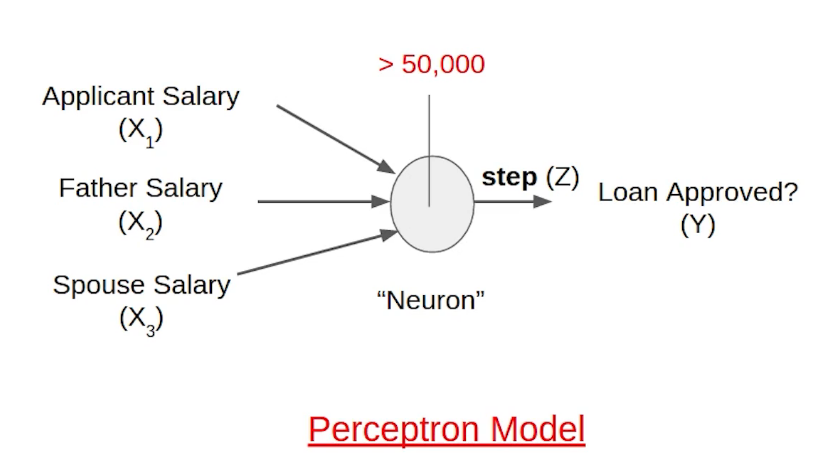## End Notes

In this article, we saw how a perceptron model works. It takes in multiple features like applicant salary, father salary, etc. as input and checks whether the sum of these, which is the total income of the household, exceeds the threshold or not. If it does only then it will give an output as one, which means the loan should be approved, otherwise the step function will give an output as zero.

If you are looking to kick start your Data Science Journey and want every topic under one roof, your search stops here. Check out Analytics Vidhya’s Certified AI & ML BlackBelt Plus Program.

I hope this article helps you understand perceptron. In case you have any queries, feel free to reach out in the comments below.